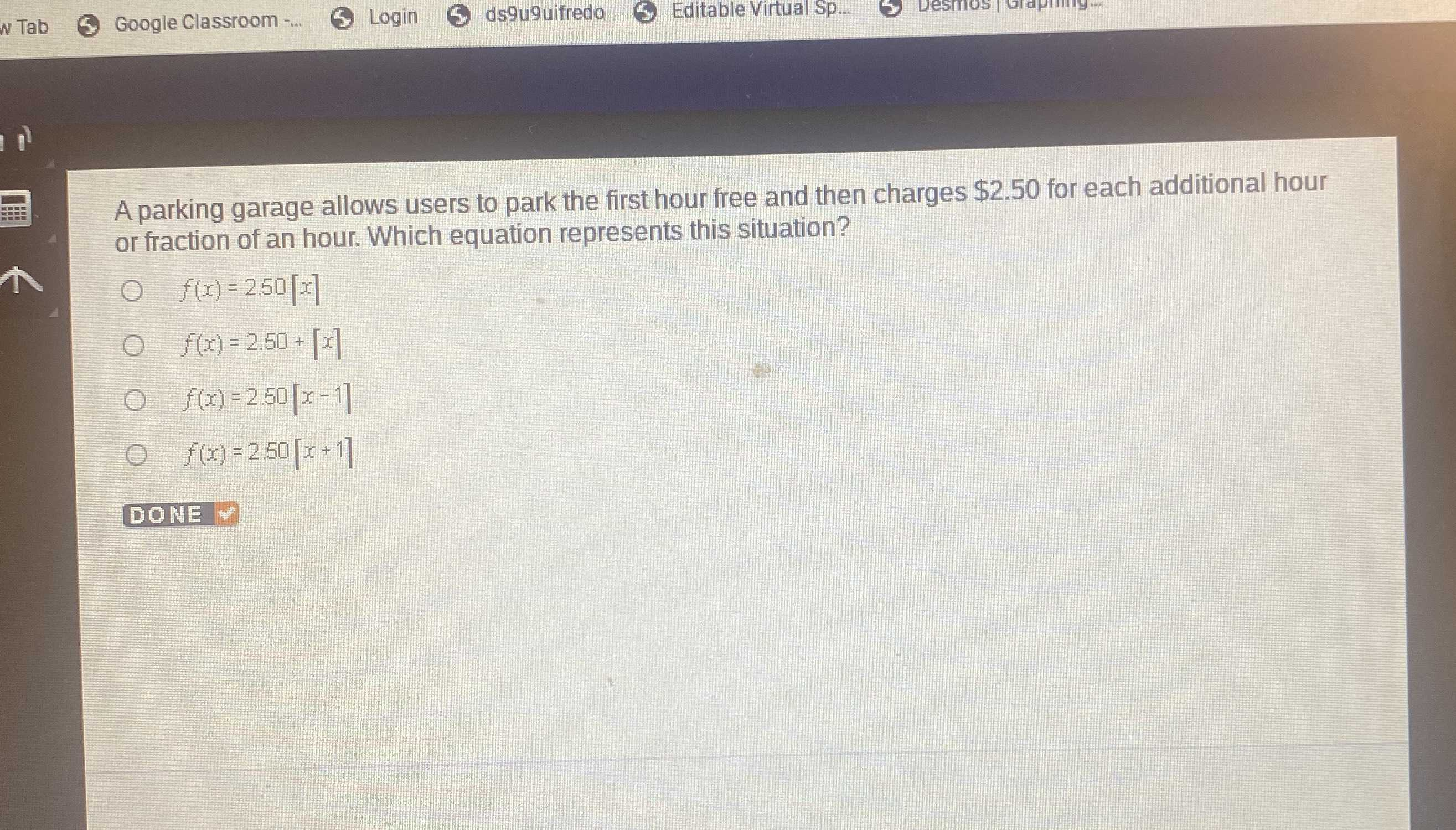### Still have math questions?

Algebra
QuestionA parking garage allows users to park the first hour free and then charges $$\ 2.50$$ for each additional hour or fraction of an hour. Which equation represents this situation?

$$f ( x ) = 2.50 [ x ]$$

$$f ( x ) = 2.50 + [ x ]$$

$$f ( x ) = 2.50 [ x - 1 ]$$

$$f ( x ) = 2.50 [ x + 1 ]$$